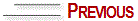# LOCATION OF THE VANISHING, VIEW AND DEPTH POINTS IN PARALLEL PERSPECTIVE

First of all, draw the three parallel and horizontal lines:

— support line

— horizon line

— distance line.

Work to a scale and perform the following operations:

A) — Draw a perfectly geometric square on the support line with the vertical and horizontal lines in parallel.

B) — Locate the vanishing and view points as follows: find the middle of the square and with a vertical line starting at the support line and going up to the horizon line (which is the viewer’s view) you will find where to mark the vanishing point. From the support line, starting at the same vertical line downwards, determine the visual distance of 17cm and locate the view point. Work always to a scale. 17cm is equal to 8.5mm in 1:20 scale.

C) — Now sketch a diagonal within the square, from one vertex to the other. Then, sketch a line parallel to this diagonal; from the view point to the distance line (we do not construct the square out of focus in order to facilitate the process of carrying out the structure). From this point, sketch a perpendicular line up to the horizon line and you will find the depth point. This operation is shown in figure nº 7.

After obtaining the vanishing, view and depth points, you will construct a flat surface to draw in perspective.

Note in figure nº 8 that the distance between the view point and the distance line is always equal to the distance between the vanishing point and the depth point. Therefore, in order to work on parallel perspective it will not be necessary to perform the whole operation shown in figure nº 7 to find the three points. You have only to place the depth point at the horizon line, far from the vanishing point in the same proportion that the view point is located at the distance line.© Yvonne Tessuto Tavares - All rights reserved